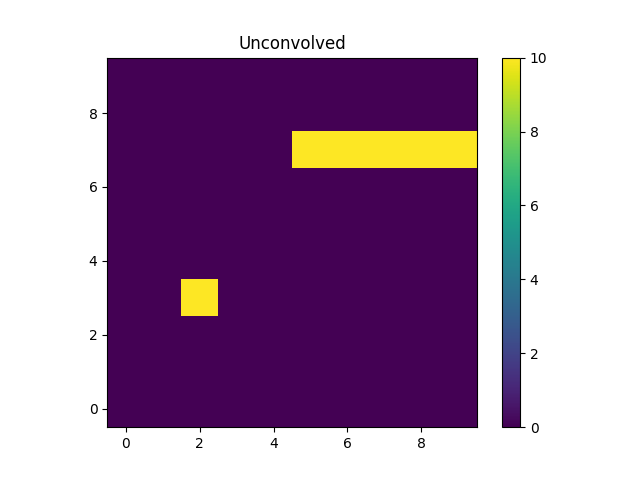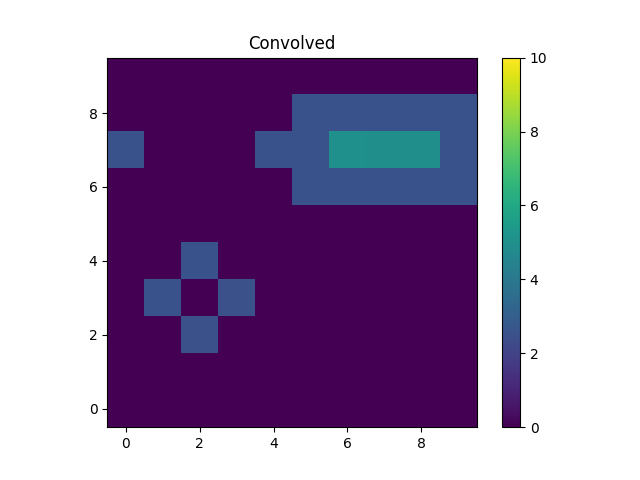# Convolution

A convolution model requires both the evaluation grid and the data to convolve. Examples include using a point-spread function (PSF) model to modify a two-dimensional model to account for blurring due to the instrument (or other sources, such as the atmosphere for ground-based Astronomical data sets), or the redistribution of the counts as a function of energy as modelled by the RMF when analyzing astronomical X-ray spectra.

Note

In the following documentation we are going to use the term “convolution” to refer to any model that requires the data from another model, such as a model that reprocesses emission into another passband or that renormalises the data, even if it does not match the meaning of convolution.

## Creating a convolution kernel

For convolution-style models, there are normally two steps to create a model component:

1. create an instance of the convolution kernel

2. apply the instance to the model components

The convolution kernel is not an instance of `sherpa.models.model.ArithmeticModel`, and so can not be evaluated by passing it the data grid. Instead, the kernel is used to wrap another model expression, normally done by passing the model to be wrapped as the argument to the model, and the result of this is then a model that can be evaluated on the data grid.

Note

Evaluating the kernel - such as `psf` in the example below - with a grid will raise an error like:

```ModelErr: attempted to create ArithmeticFunctionModel from non-callable object of type ndarray
```

## One-dimensional convolution with a PSF

In this example we want to convolve a model by the “kernel” [5, 10, 3, 2], which requires creating a `sherpa.data.Data1D` instance to store the data, and then pass it to `sherpa.instrument.PSFModel`:

```>>> import numpy as np
>>> from sherpa.data import Data1D
>>> from sherpa.instrument import PSFModel
>>> k = np.asarray([5, 10, 3, 2])
>>> x = np.arange(k.size)
>>> kernel = Data1D('kdata1', x, k)
>>> psf1 = PSFModel('psf1', kernel=kernel)
>>> print(psf1)
psf1
Param        Type          Value          Min          Max      Units
-----        ----          -----          ---          ---      -----
psf1.kernel  frozen       kdata1
psf1.norm    frozen            1            0            1
```

To show how this model works we will use it to convolve a `Box1D` model (this is chosen since it has sharp edges and so the convolution is more obvious).

```>>> from sherpa.models.basic import Box1D
>>> point = Box1D('pt')
>>> point.xlow = 9.5
>>> point.xhi = 10.5
>>> print(point)
pt
Param        Type          Value          Min          Max      Units
-----        ----          -----          ---          ---      -----
pt.xlow      thawed          9.5 -3.40282e+38  3.40282e+38
pt.xhi       thawed         10.5 -3.40282e+38  3.40282e+38
pt.ampl      thawed            1           -1            1
```

The convolution case is created by applying the `psf1` model to the `point` model (the 2D example below shows an example of applying a kernel to a composite model):

```>>> convolved = psf1(point)
>>> print(convolved)
psf1(pt)
Param        Type          Value          Min          Max      Units
-----        ----          -----          ---          ---      -----
pt.xlow      thawed          9.5 -3.40282e+38  3.40282e+38
pt.xhi       thawed         10.5 -3.40282e+38  3.40282e+38
pt.ampl      thawed            1           -1            1
```

The model can be evaluated both before and after convolution, by passing it the data grid. Unlike normal model evaluation the `PSFModel` class requires that its `fold()` model be called before evaluation. This method pre-calculates terms needed for the convolution (which is done using a fourier transform), and so needs the grid over which it is to be applied. This is done by passing in a `Data` instance (in this case the Y data in the `Data1D` instance is not used so is set to zero):

```>>> x = np.arange(6, 15)
>>> blank = Data1D('blank', x, np.zeros(x.size))
>>> psf1.fold(blank)
```

With this out of the way, we can compare the convolved to un-convolved resuls:

```>>> y1 = point(x)
>>> y2 = convolved(x)
>>> for z in zip(x, y1, y2):
...     print("x: {:2d}  y: {:.0f}  convolved: {:.2f}".format(*z))
x:  6  y: 0  convolved: 0.00
x:  7  y: 0  convolved: 0.00
x:  8  y: 0  convolved: 0.00
x:  9  y: 0  convolved: 0.25
x: 10  y: 1  convolved: 0.50
x: 11  y: 0  convolved: 0.15
x: 12  y: 0  convolved: 0.10
x: 13  y: 0  convolved: 0.00
x: 14  y: 0  convolved: 0.00
```

The `PSFModel` instance has automatically selected the largest pixel in the kernel as the center (in this case the second element, 10), and has automatically re-normalized the kernel. The parameters of the convolution kernel (in this case `psf1`) can be changed to control the behavior.

```>>> print(psf1)
psf1
Param        Type          Value          Min          Max      Units
-----        ----          -----          ---          ---      -----
psf1.kernel  frozen       kdata1
psf1.size    frozen            4            4            4
psf1.center  frozen            2            2            2
psf1.norm    frozen            1            0            1
```

Note

The model parameters for the convolution kernel have changed since earlier, as the use of the `fold()` method has added new parameters (in this case the `size` and `center` parameters).

## Two-dimensional convolution with a PSF

The `sherpa.astro.instrument.PSFModel` class augments the behavior of `sherpa.instrument.PSFModel` by supporting images with a World Coordinate System (WCS). For this example we do not need this capability and so use the `sherpa.instrument.PSFModel` class directly.

### Including a PSF in a model expression

The “kernel” of the PSF is the actual data used to represent the blurring, and can be given as a numeric array or as a Sherpa model. In the following example a simple 3 by 3 array is used to represent the PSF, but it first has to be converted into a `Data2D` object (this is similar to the steps needed in the 1D case above):

```>>> from sherpa.data import Data2D
>>> from sherpa.instrument import PSFModel
>>> k = np.asarray([[0, 1, 0], [1, 0, 1], [0, 1, 0]])
>>> yg, xg = np.mgrid[:3, :3]
>>> kernel = Data2D('kdata', xg.flatten(), yg.flatten(), k.flatten(),
...                 shape=k.shape)
>>> psf = PSFModel(kernel=kernel)
>>> print(psf)
psfmodel
Param        Type          Value          Min          Max      Units
-----        ----          -----          ---          ---      -----
psfmodel.kernel frozen        kdata
psfmodel.norm frozen            1            0            1
```

As shown below, the data in the PSF is renormalized so that its sum matches the `norm` parameter, which here is set to 1.

The following example sets up a simple model expression which represents the sum of a single pixel and a line of pixels, using `Box2D` for both.

```>>> from sherpa.models.basic import Box2D
>>> pt = Box2D('pt')
>>> pt.xlow, pt.xhi = 1.5, 2.5
>>> pt.ylow, pt.yhi = 2.5, 3.5
>>> pt.ampl = 8
>>> box = Box2D('box')
>>> box.xlow, box.xhi = 4, 10
>>> box.ylow, box.yhi = 6.5, 7.5
>>> box.ampl = 10
>>> unconvolved_mdl = pt + box
>>> print(unconvolved_mdl)
(pt + box)
Param        Type          Value          Min          Max      Units
-----        ----          -----          ---          ---      -----
pt.xlow      thawed          1.5 -3.40282e+38  3.40282e+38
pt.xhi       thawed          2.5 -3.40282e+38  3.40282e+38
pt.ylow      thawed          2.5 -3.40282e+38  3.40282e+38
pt.yhi       thawed          3.5 -3.40282e+38  3.40282e+38
pt.ampl      thawed            8 -3.40282e+38  3.40282e+38
box.xlow     thawed            4 -3.40282e+38  3.40282e+38
box.xhi      thawed           10 -3.40282e+38  3.40282e+38
box.ylow     thawed          6.5 -3.40282e+38  3.40282e+38
box.yhi      thawed          7.5 -3.40282e+38  3.40282e+38
box.ampl     thawed           10 -3.40282e+38  3.40282e+38
```

Note

Although Sherpa provides the `Delta2D` class, it is suggested that alternatives such as `Box2D` be used instead, since a delta function is very sensitive to the location at which it is evaluated. However, including a `Box2D` component in a fit can still be problematic since the output of the model does not vary smoothly as any of the bin edges change, which is a challenge for the optimisers provided with Sherpa.

Rather than being another term in the model expression - that is, an item that is added, subtracted, multiplied, or divided into an existing expression - the PSF model “wraps” the model it is to convolve. This can be a single model or - as in this case - a composite one:

```>>> convolved_mdl = psf(unconvolved_mdl)
>>> print(convolved_mdl)
psfmodel((pt + box))
Param        Type          Value          Min          Max      Units
-----        ----          -----          ---          ---      -----
pt.xlow      thawed          1.5 -3.40282e+38  3.40282e+38
pt.xhi       thawed          2.5 -3.40282e+38  3.40282e+38
pt.ylow      thawed          2.5 -3.40282e+38  3.40282e+38
pt.yhi       thawed          3.5 -3.40282e+38  3.40282e+38
pt.ampl      thawed            8 -3.40282e+38  3.40282e+38
box.xlow     thawed            4 -3.40282e+38  3.40282e+38
box.xhi      thawed           10 -3.40282e+38  3.40282e+38
box.ylow     thawed          6.5 -3.40282e+38  3.40282e+38
box.yhi      thawed          7.5 -3.40282e+38  3.40282e+38
box.ampl     thawed           10 -3.40282e+38  3.40282e+38
```

This new expression can be treated as any other Sherpa model, which means that we can apply extra terms to it, such as adding a background component that is not affected by the PSF:

```>>> from sherpa.models.basic import Const2D
>>> bgnd = Const2D('bgnd')
>>> bgnd.c0 = 0.25
>>> print(convolved_mdl + bgnd)
(psfmodel((pt + box)) + bgnd)
Param        Type          Value          Min          Max      Units
-----        ----          -----          ---          ---      -----
pt.xlow      thawed          1.5 -3.40282e+38  3.40282e+38
pt.xhi       thawed          2.5 -3.40282e+38  3.40282e+38
pt.ylow      thawed          2.5 -3.40282e+38  3.40282e+38
pt.yhi       thawed          3.5 -3.40282e+38  3.40282e+38
pt.ampl      thawed            8 -3.40282e+38  3.40282e+38
box.xlow     thawed            4 -3.40282e+38  3.40282e+38
box.xhi      thawed           10 -3.40282e+38  3.40282e+38
box.ylow     thawed          6.5 -3.40282e+38  3.40282e+38
box.yhi      thawed          7.5 -3.40282e+38  3.40282e+38
box.ampl     thawed           10 -3.40282e+38  3.40282e+38
bgnd.c0      thawed         0.25 -3.40282e+38  3.40282e+38
```

In the following this extra term (`bgnd`) is not included to simplify the comparison between the unconvolved and convolved versions.

### Evaluating a model including a PSF

The PSF-convolved model can be evaluated - in most cases - just as is done for ordinary models. That is by supplying it with the grid coordinates to use. However, the need to convolve the data with a fixed grid does limit this somewhat.

For this example, a grid covering the points 0 to 9 inclusive is used for each axis (with a unit pixel size), which means that the unconvolved model can be evaluated with the following:

```>>> yg, xg = np.mgrid[:10, :10]
>>> xg1d, yg1d = xg.flatten(), yg.flatten()
>>> m1 = unconvolved_mdl(xg1d, yg1d).reshape(xg.shape)
```

An easier alternative, once the PSF is included, is to create an empty dataset with the given grid (that is, a dataset for which we do not care about the dependent axis), and use the `eval_model()` method to evaluate the model (the result for `m1` is the same whichever approach is used):

```>>> blank = Data2D('blank', xg1d, yg1d, np.ones(xg1d.shape), xg.shape)
>>> m1 = blank.eval_model(unconvolved_mdl).reshape(xg.shape)
```The “point source” is located at `x = 2, y = 3` and the line starts at `x=5` and extends to the end of the grid (at `y=7`).

Note

In this example the image coordinates were chosen to be the same as those drawn by matplotlib. The `extent` parameter of the `imshow` call can be used when this correspondance does not hold.

As with the 1D case, the `fold()` method must be called before evaluation.

```>>> psf.fold(blank)
>>> m2 = blank.eval_model(convolved_mdl).reshape(xg.shape)
```The kernel used redistributes flux from the central pixel to its four immediate neighbors equally, which is what has happened to the point source at `(2, 2)`. The result for the line is to blur the line slightly, but note that the convolution has “wrapped around”, so that the flux that should have been placed into the pixel at `(10, 7)`, which is off the grid, has been moved to `(0, 7)`.

Note

If the `fold` method is not called then evaluating the model will raise the following exception:

```PSFErr: PSF model has not been folded
```

Care must be taken to ensure that `fold` is called whenever the grid has changed. This suggests that the same PSF model should not be used in simultaneous fits, unless it is known that the grid is the same in the multiple datasets.

### The PSF Normalization

Since the `norm` parameter of the PSF model was set to 1, the PSF convolution is flux preserving, even at the edges thanks to the wrap-around behavior of the fourier transform. This can be seen by comparing the signal in the unconvolved and convolved images, which are (to numerical precision) the same:

```>>> m1.sum()
58.0
>>> m2.sum()
58.0
```

The use of a fourier transform means that low-level signal will be found in many pixels which would expect to be 0. For example, looking at the row of pixels at `y = 7` gives:

```>>> m2
array([2.50000000e+00, 1.73472348e-16, 5.20417043e-16, 4.33680869e-16,
2.50000000e+00, 2.50000000e+00, 5.00000000e+00, 5.00000000e+00,
5.00000000e+00, 2.50000000e+00])
```